# Convolution

Categories:

Updated:

We convolve filters to input and activate it to get next layer.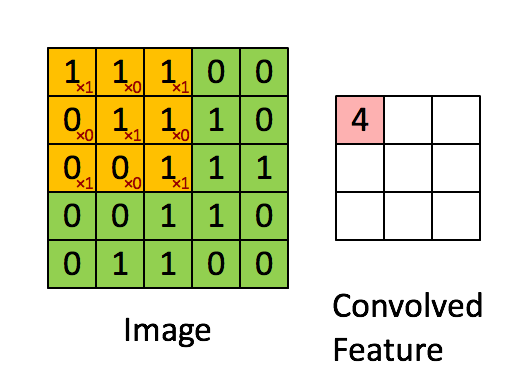In the above gif, we are convolving 3x3 filter to an 2-dimensional input with. Our filter is

We multiply filter with the left-top subset of the input(same size as filter) element-wise, slide it to right/down and repeat until we’ve arrived at the right-bottom.

# Channels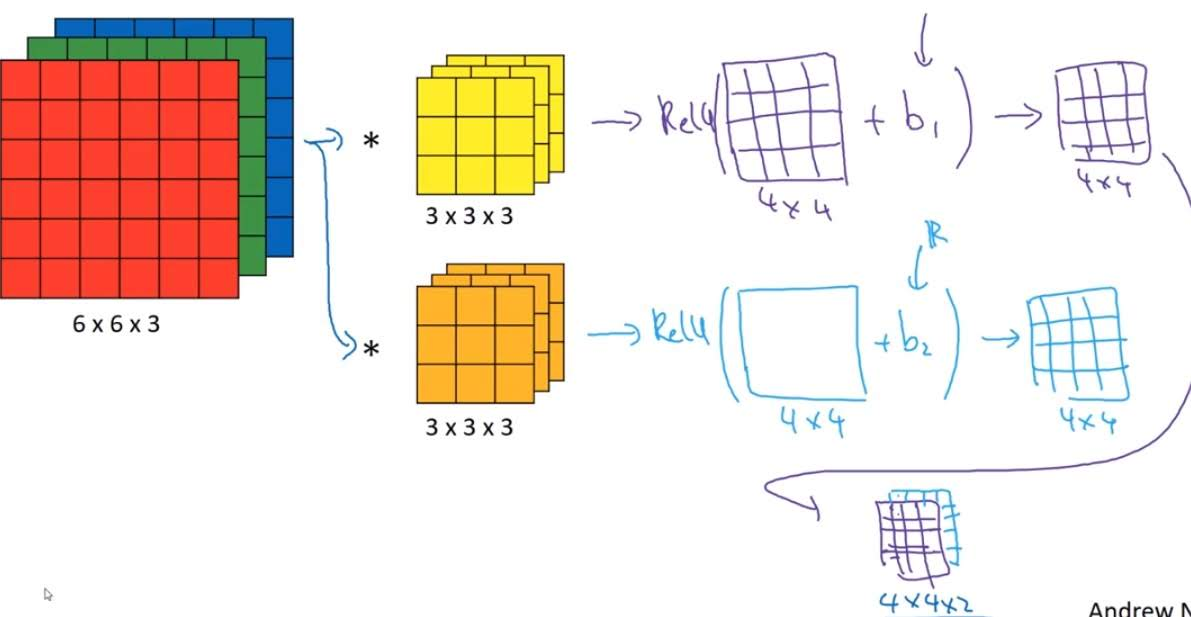In the above image, input is a 6x6 size image. With RGB, there are three channels in each pixel so the input shape is 6x6x3. For example, if the left top pixel is red, first channel of that pixel would be 255, second 0, third 0. If the image is black and white, it would only have 1 channel.

Number of channels of filter applied to this input should also be 3.

Also, you can apply multiple filters to the input. Number of filters you apply would be the next layer’s number of channels.

When doing convolution, pixels in the border contributes less than the inner pixels. By adding pixels of value 0 around the border of the input, we can solve this problem.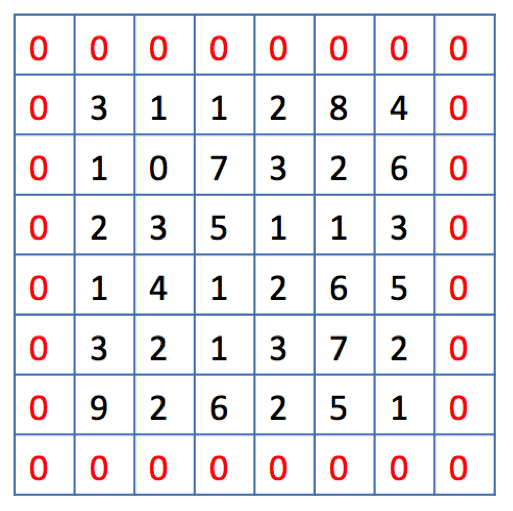We call it same convolution when we pad so that the output size is the same as the input size. Also convolution without padding is called valid convolution.

# Stride

The number of pixels you slide filter is called stride.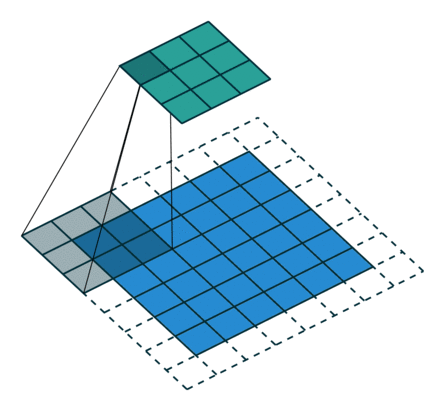In the above example, we are convolving 3x3 filter to a 6x6 input with padding 1 and stride 2.

# Shapes

## Notations

If layer $l$ is a convolution layer with activation shape $n_H^{[l]} \times n_W^{[l]} \times n_c^{[l]}$;

$f^{[l]}$: filter size

$p^{[l]}$: padding

$s^{[l]}$: stride

$n_f^{[l]}$: number of filters

## Shapes

Each filter: $f^{[l]}\times f^{[l]}\times n_c^{[l]}$

Weights: $f^{[l]} \times f^{[l]} \times n_c^{[l]} \times n_f^{[l]}$

Bias: $n_f^{[l]}$

Output: $n_H^{[l+1]} \times n_W^{[l+1]} \times n_f^{[l]}$, where $n_H^{[l+1]}=\lfloor \frac{n_H^{[l]} + 2p^{[l]} - f^{[l]}}{s^{[l]}} + 1 \rfloor$ (same with $W$)

With multiple examples, $m$ would be multiplied to the front of the shapes. For example, $A^{[l]}: m \times n_H^{[l]} \times n_W^{[l]} \times n_c^{[l]}$

# Convolution as Feature Detector

Convolution filters act as feature detector of an image.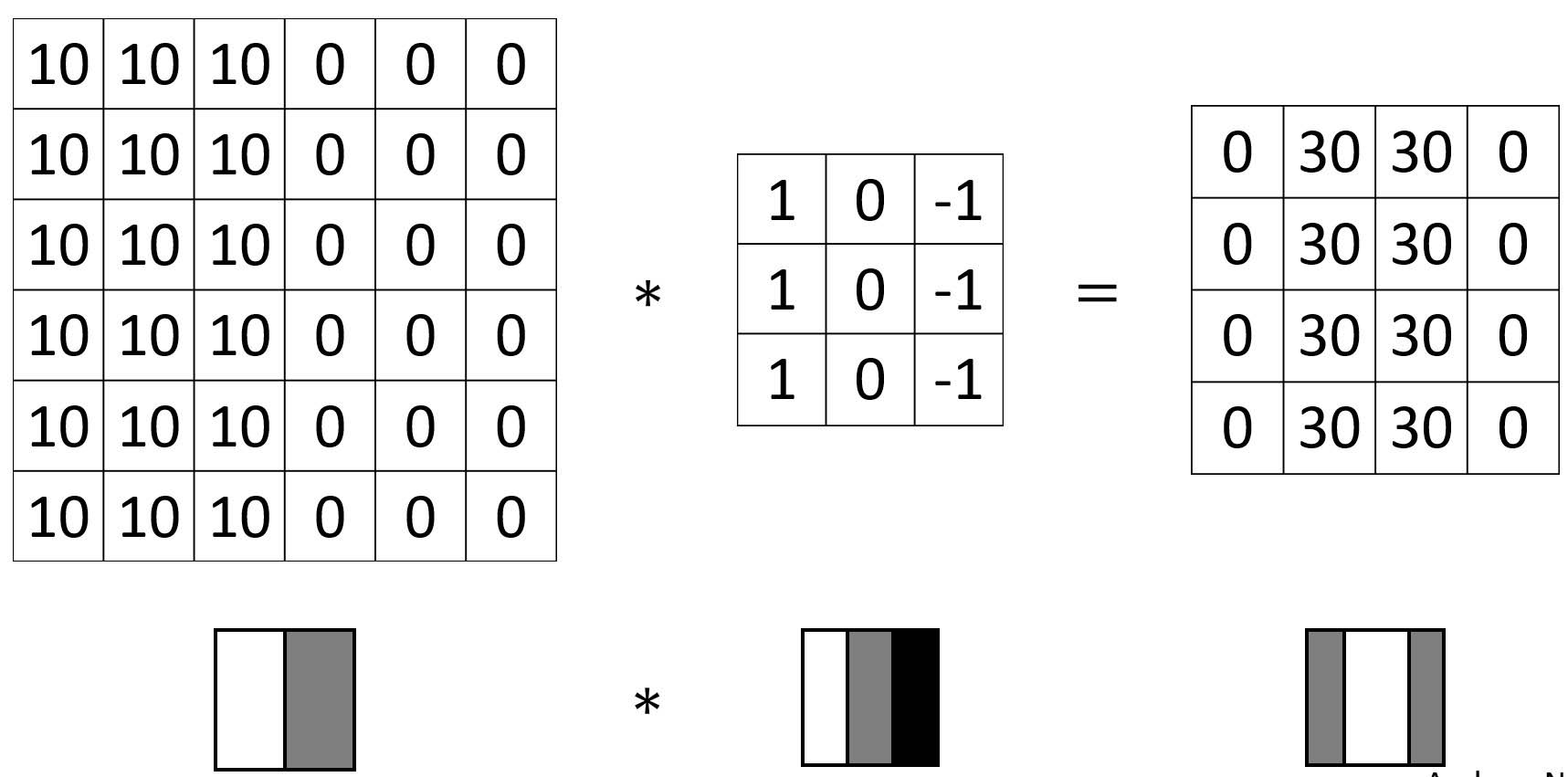For example, in the above image, 3x3 filter detects vertical border of the image.

# Why does Convolution Suit Vision Problems?

### 1. Parameter sharing

A feature detector (such as a vertical edge detector) that’s useful in one part of the image is probably useful in another part of the image.

### 2. Sparsity of connections

In each layer, each output value depends only on a small number of inputs.

### 3. Translation invariance

With image with a cat on the left and image with a cat on the right, CNN would yield similar results.

Categories: